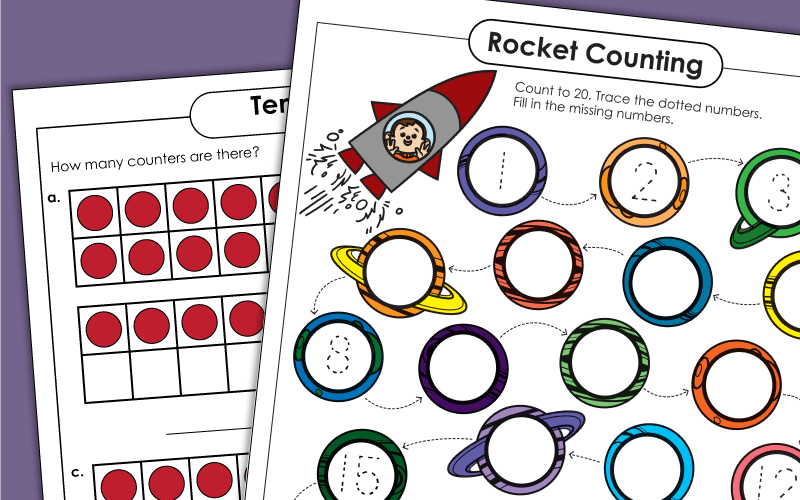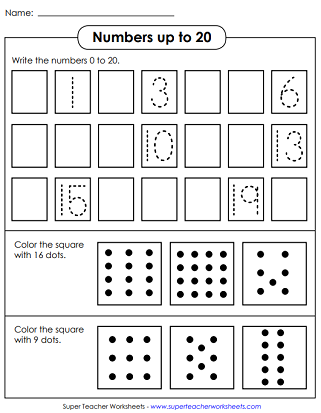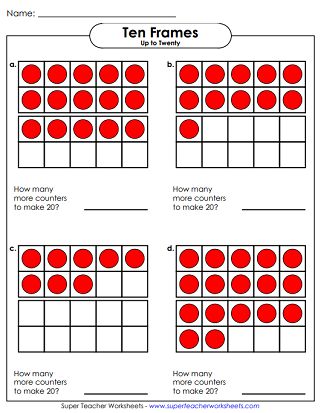# Numbers and Counting (Up to 20)

With the worksheets on this page, kids can learn to recognize and count numbers up to twenty. Many of these worksheets align with common core standards K.CC.3 and K.CC.4.## Number Eleven

Trace and print the number 11 on the primary-ruled lines. Then draw 11 stars in the box at the bottom of the page.
Find the place value blocks that show the number 11. Tell whether 11 is odd or even. Draw 11 tally marks. Show 11 on the ten-frames, and complete the number line.

## Number Twelve

Trace and print the number twelve. Then draw 12 hearts in the box.
Tell how many tens and how many ones are in the special number. Show 12 on a 10-frame. Make 12 tallies. Complete the number line.

## Number Thirteen

After writing the number 13 on the lines provided, students will draw 13 triangles at the bottom of the page.
Trace the word "thirteen." Then draw 13 tallies. Make 13 on the 10-frame. Fill in the numbers before and after 13 on the number line.

## Number Fourteen

Write the number fourteen on the lines, and then draw 14 ovals in the box.
Count to 14. Tell how many 10s and 1s are in this number. Draw 14 dots. Find the base-10 blocks that show 14.

## Number Fifteen

Trace and print the number fifteen. Then draw 15 diamonds.
Tell whether 15 is odd or even. Find the place value blocks for 15. Make 15 colored dots with crayons. Tell how many ones and how many tens are in this number.

## Number Sixteen

Count sixteen baseballs. Trace and print the number 16. Draw sixteen smile faces.
Trace and write 16. Make 16 on the double 10-frame. Complete the number line. Tell how many 10s and 1s in this number.

## Number Seventeen

First, write the number 17. Then count 17 strawberries. Then make 17 checkmarks.
How many tens and ones in the special number? Fill in the empty boxes on the number line.

## Number Eighteen

There are 18 rulers in the picture can you count them all? Write the number 18 on the lines. Draw 18 circles in the box at the bottom.
Determine which set of base-10 blocks represents 18. Tell whether the secret number is odd or even. Draw 18 tally marks.

## Number Nineteen

Count 19 seashells. Write the number 19. Then draw 19 spirals.
Tell if 19 is an even or odd number. Then draw 19 dots. After that, make 19 tallies. Then circle the base-10 blocks that represent 19.

## Number Twenty

Count twenty balloons and make a sketch with twenty zigzag lines in it.
How many 10s and 1s are in the number 20? Make 20 with tally marks. Put 20 dots on the 10-frames. Circle the place value blocks that show 20.

## Counting Up to 20

Write numbers from 0 through 20. Then select the boxes with the correct number of dots.
Pre-K and Kindergarten
Write the missing numbers in the circles from 1 through 20 and trace the numbers that are provided on this printable counting worksheet.
Count from 1 to 20. Fill in the missing numbers on the number line.
Kindergarten
Count the pennies (up to 14 cents) and write the amount of money shown.
Count the tens and ones blocks to find the number. Glue the matching number from the bottom of the page to the correct box.

## Double Ten Frames

Tell how many counters are pictured in each double ten-frame. Practice counting up to 20.
Draw the correct number of circles in each double ten-frame.
Use this ten frame template for counting, adding, or subtracting.
Tell how many more counters are needed to make 20.

## Dot-to-Dot

Count from 1 up to 20. Connect the dots to make an elephant picture.

## Skip Counting

On this printable worksheet, student will practice skip counting by 2s up to 18.

## Word Wall

This word wall card set has numbers and words. The cards should also fit in classroom pocket charts.
Numbers and Counting (Up to 10)

Learn to recognize numbers and count up to ten with these printables.

Numbers and Counting (Up to 30)

These worksheets focus on number recognition and counting up to 30.

Ten-Frame Worksheets

On this page, we have five-frame, ten-frame, and double ten-frame activities and worksheets.

Dot-to-Dot Printables

Practice alphabet and counting skills with these dot-to-dot puzzles.

## Pictures of WorksheetsMy Account
Site Information